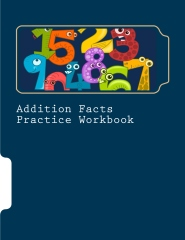# Math Facts Practice

### Found a problem? Check here.We have facts practice workbooks that you can print or buy.

Xtramath.org  — to drill all of your facts (starts with addition, then moves to subtraction, multiplication and finally, division)

Math 1 — Addition  (facts 0 – 5)

Balloon Pop

Central Park

Coin Craze  Type in 7, 8, 9, or 10 and click Add.

Empire State Building

End of the World

Flashcards  change maximums to 5

Martian Math  Choose Facts to 5 and Add.

Robot Addition – Choose 1 – 5.

Scuba Diver  Choose Facts to 5 and Add.

Sharks

Soft Serve Shop  Choose Facts to 5 and Add.

Space Slime  Choose Facts to 5 and Add.

Math 1 — Subtraction  (facts 0 – 5)

Asteroid Adventure  Choose Facts to 5 and Subtract.

Baseball  Subtraction, easy

Bubbles

Coin Craze   Type in 2, 3, 4, or 5 and click Subtract.

Figure It  Subtraction Level 1

Fishing

Flashcards

Island Chase  Choose Custom Content. Type 10 in the second box.

Martian Math  Choose Facts to 5 and Subtract.

Math Test

Minus Mission  Type 10 in the second box.

Robot Addition – Choose the big Less Than button at the top and 1 – 5.

Save the World

Scuba Diver  Choose Facts to 5 and Subtract.

Soft Serve Shop  Choose Facts to 5 and Subtract.

Space Slime  Choose Facts to 5 and Subtract.

Math 2 – Addition  (facts 0 – 10)

Alien Blaster  Click “play” and read the directions. On one page it will ask you what number range you want to add. Leave the first number as 1. Change the second number to 10.

Bridge Builder  Build all three bridges by dragging the blocks. When you get bigger blocks, click on smaller blocks that add up to that number and then on Trade. For example, if you get a ten block, then you could click on a 7 and 3 block or a 4 and 6 block and then click on trade. Your goal is to use all the biggest blocks.

Coin Craze  Type in 11, 12, 13, 14, or 15 and click Add.

End of the World

Figure It!  Choose Addition and Level 2.

Hidden Picture  Click on the problem that equals the answer.

Martian Math  Choose Facts to 10 and Add.

Math Challenge  Click on addition under level one. Work until the timer finishes.

Scuba Diver  Choose Facts to 10 and Add.

Soft Serve Shop  Choose Facts to 10 and Add.

Space Slime  Choose Facts to 10 and Add.

Tennis Facts

War – The classic card game – Choose Math Options, Arithmetic, Addition No. Add the card values together. There are a few tricky ones you’ll have to figure out.

Math 2 – Subtraction  (facts 0 – 10)

Asteroid Adventure  Choose Facts to 10 and Subtract.

Balloons

Coin Craze  Type in 7 and click subtract. (You can try other numbers under 10 as well.)

Deep Dive  Choose 3, 4, 5, 6, 7, 8 and 9.

End of the World

Figure It  Subtraction Level 2

Martian Math  Choose Facts to 10 and Subtract.

Puzzle Subtraction  ? – ? = ?  Click on the checkmark. Say the answer and click on the card. It will show you the correct answer tiny in the corner.

Scuba Diver  Choose Facts to 10 and Subtract.

Soft Serve Shop  Choose Facts to 10 and Subtract.

Space Slime  Choose Facts to 10 and Subtract.

Trimathalon

World Cup  Choose subtraction.

Multiplication

Farm Escape

Figure It  Multiplication Level 2

Hit the Button

Scuba Diver

Martian Math

Multiplication.com

Sky Diver

Soft Serve Shop

Space Slime

War – The classic card game – Choose Math Options, Arithmetic, Multiplication, No. Multiply the values of the two cards. There are a few tricky ones to figure out…

Division

Farm Escape

Figure It  Division Level 2